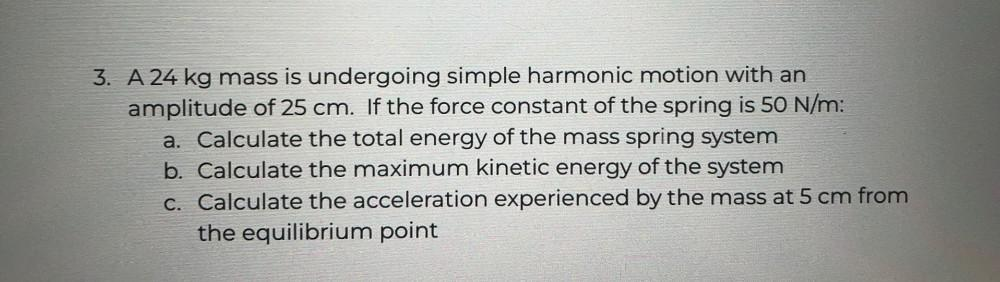Question:

# 3. A 24 kg mass is undergoing simple harmonic motion with an amplitude of 25 cm. If the force constant of the spring is 50 N/m:3. A 24 kg mass is undergoing simple harmonic motion with an amplitude of 25 cm. If the force constant of the spring is 50 N/m: a. Calculate the total energy of the mass spring system b. Calculate the maximum kinetic energy of the system C. Calculate the acceleration experienced by the mass at 5 cm from the equilibrium point